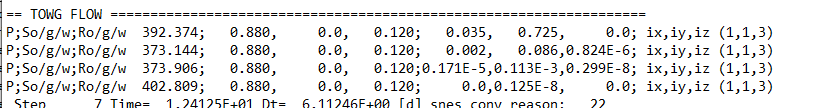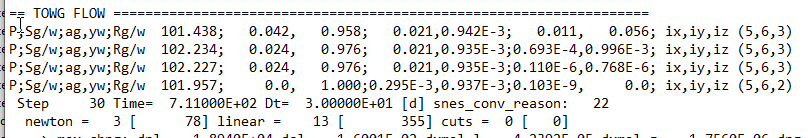# The SOLUTION_MONITOR Tool¶

The SOLUTION_MONITOR is an advanced option that may be selected in the OUTPUT section block, for example:

OUTPUT
SOLUTION_MONITOR
END


The purpose of this tool is to add output to help in identifying the cells having the most difficulty converging, throughout the run.

Output will be added to the run’s .out file, for each step and nonlinear iteration, giving information about the cell with the current greatest residual. Note that all residual values discussed here are the absolute values.

## Output in Black Oil Mode¶

Here is an example of the output for one step in a black oil run:Each row corresponds to the state after one nonlinear iteration (except the first row, which is the state at the start of the step before any iterations). The key P;So/g/w;Ro/g/w indicates that the following columns will display the pressure, then saturation of oil, water and gas, then the residual in the oil water and gas conservation equations; in the cell with the greatest resdiual. Then the key ix,iy,iz indicates that the coordinates of that cell will be displayed next.

As an example, considering the second line of the above, we see that after one noninear iteration the cell with the greatest residual is located at (1,1,3), has pressure 373.144, oil saturation 0.880, 0 gas saturation, and water saturation 0.120. It has residuals of 0.001, 0.086, and 0.824E-6 in the oil, gas and water equations respectively.

## Output in Gas-Water Mode¶

Here is an example of the output for one step in a gas-water run:Each row corresponds to the state after one nonlinear iteration (except the first row, which is the state at the start of the step before any iterations). The key P;Sg/w;ag,yw;Rg/w indicates that the following columns will display the pressure, then saturation water and gas, then the concentration of gas in the aqueous phase, concentration of water in the vapour phase, then the residuals in the gas and water conservation equations; in the cell with the greatest residual. Then the key ix,iy,iz indicates that the coordinates of that cell will be displayed next.

As an example, considering the second line of the above, we see that after one noninear iteration the cell with the greatest residual is located at (5,6,3), has pressure 102.235, 0.024 gas saturation and 0.976 water saturation. It has gas in aqueous concentration 0.021, and water in vapour concentration 0.935E-3. It has residuals of 0.693E-4 and 0.9963E-3 in the gas and water equations respectively.